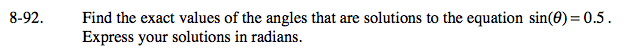Home > A2C > Chapter 8 > Lesson 8.1.6 > Problem8-92

8-92.

Find the exact values of the angles that are solutions to the equation sin(θ) = 0.5. Express your solutions in radians. 8-92 HW eTool (Desmos). Homework Help ✎Draw a unit circle and a triangle with the opposite side of 0.5 and hypotenuse 1.
Since the short leg is half the hypotenuse, this is a 30°-60°-90° triangle. θ = 30°.

Reflect the triangle across the y-axis. If 30° is the reference angle, what is the circular angle?
Notice that θ = 150° as well.

Convert both angles to radians. Recall that 180° is π radians. What fraction of 180° is 150°?

Every time you go around the circle, you will come back to these points, so there are an infinite number of solutions to this equation.
A new solution occurs every 360° or 2π radians.

$\frac{\pi}{6} \pm 2\pi, \frac{5 \pi}{6} \pm 2 \pi$

Use the eTool below to view the solution as a graph.
Click the link at right for the full version of the eTool: A2C 8-92 HW eTool